# JIT in ClickHouse

Maksim, developer of ClickHouse.

## Query processing

1. Vectorized query execution

2. Runtime code generation

ClickHouse uses both

## Query execution plan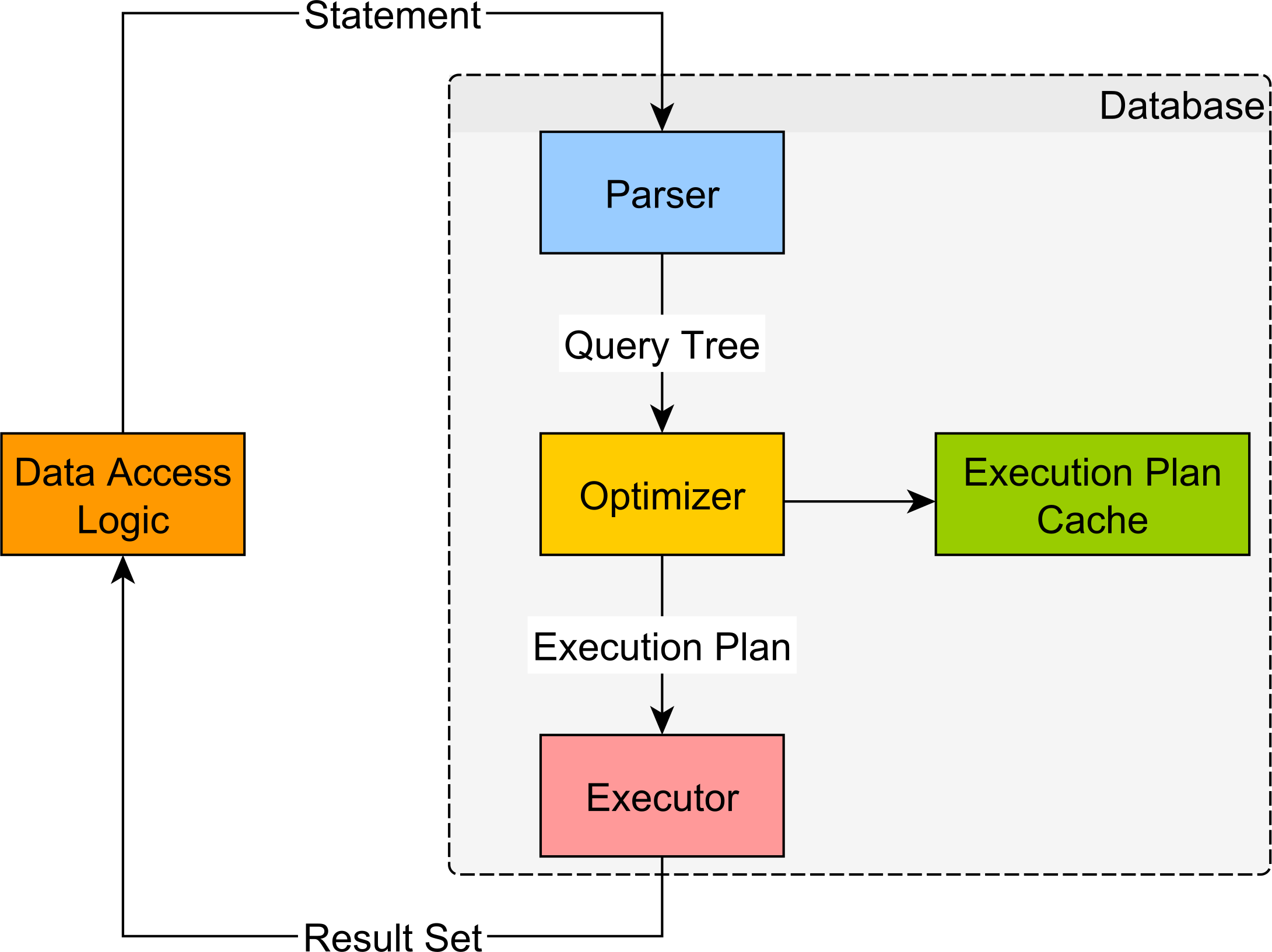## Query execution plan

```EXPLAIN PIPELINE SELECT a + b + c FROM test_table ┌─explain───────────────────┐ │ (Expression) │ │ ExpressionTransform │ │ (SettingQuotaAndLimits) │ │ (ReadFromStorage) │ │ TinyLog 0 → 1 │ └───────────────────────────┘ ```

## ExpressionTransform

Expressions are represented in DAG that has input, function, constant nodes.

`SELECT a + b + c FROM test_table;`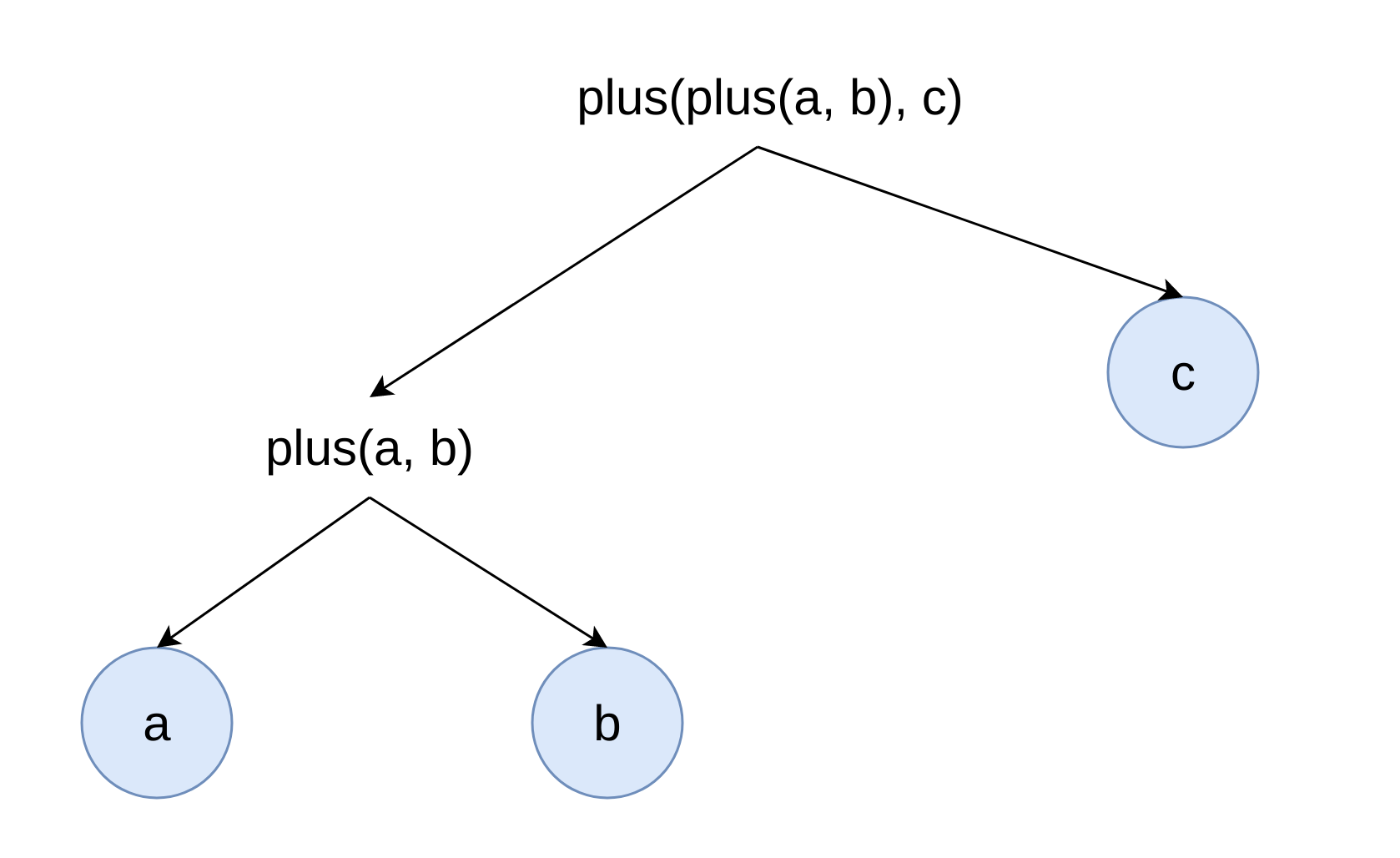## How JIT can help

1. Improve L1, L2 cache usages.

2. Less code to execute. Better usage of CPU branch predictor.

3. Eliminate indirections.

4. Multiple operations are fused in one function. More optimizations can be performed by compiler.

5. Using target CPU intructions.

## Why does it matter

```Latency Comparison Numbers
----------------------------------
L1 cache reference                           0.5 ns
Branch mispredict                            5   ns
L2 cache reference                           7   ns 14x L1 cache
Mutex lock/unlock                           25   ns
Main memory reference                      100   ns 20x L2 cache, 200x L1 cache
Compress 1K bytes with Zippy             3,000   ns        3 us
Send 1K bytes over 1 Gbps network       10,000   ns       10 us
Read 4K randomly from SSD*             150,000   ns      150 us ~1GB/sec SSD
Read 1 MB sequentially from memory     250,000   ns      250 us
Round trip within same datacenter      500,000   ns      500 us
```

## Compilation costs

JIT standard expression compilation time is around 15ms. Grows linearly with code size.

Typical compiled expression code take around 600 bytes.

## Example

`SELECT a + b + c FROM test_table;`## Example

```SET compile_expressions = 1; SELECT a + b + c FROM test_table;```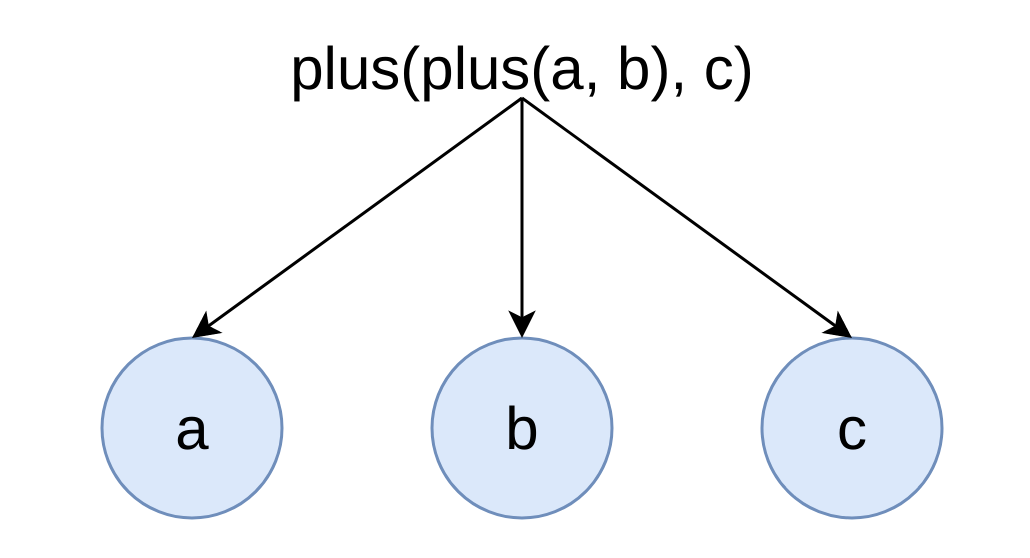## Settings

compile_expressions is true by default from ClickHouse 21.6

min_count_to_compile_expression by default is 3

compiled_expression_cache_size by default is 1 GB

## Which functions can be compiled

1. Binary operators. Example plus, minus, multiply, xor.

2. Unary operators. Example abs.

3. Logical functions. Example and, or, not.

4. Branch functions. Example if, multiIf.

5. Bit shift functions. Example bitShiftLeft

## JIT compilation examples

```SELECT count() FROM hits WHERE ((EventDate >= '2018-08-01') AND (EventDate <= '2018-08-03') AND (CounterID >= 34)) OR ((EventDate >= '2018-08-04') AND (EventDate <= '2018-08-05') AND (CounterID <= 101500)) ```

— 649 533 033 rows per second.

## JIT compilation examples

```SET compile_expressions = 1; SELECT count() FROM hits WHERE ((EventDate >= '2018-08-01') AND (EventDate <= '2018-08-03') AND (CounterID >= 34)) OR ((EventDate >= '2018-08-04') AND (EventDate <= '2018-08-05') AND (CounterID <= 101500)) ```

— 865 491 052 rows per second.
+33% performance improvement!

## JIT compilation examples

```SELECT number * 2 + number * 3 + number * 4 + number * 5 FROM system.numbers FORMAT Null ```

— 0 rows in set. Elapsed: 0.903 sec. Processed 329.23 million rows, 2.63 GB (364.78 million rows/s., 2.92 GB/s.

## JIT compilation examples

```SET compile_expressions = 1; SELECT number * 2 + number * 3 + number * 4 + number * 5 FROM system.numbers FORMAT Null ```

— 0 rows in set. Elapsed: 1.602 sec. Processed 1.89 billion rows, 15.15 GB (1.18 billion rows/s., 9.46 GB/s.)
+323% performance improvement!

## JIT compilation examples

```SET compile_expressions = 1; WITH number AS x, if(x = 1, 1, if(x = 2, 2, if(x = 3, 3, if(x = 4, 4, if(x = 5, 5, if(x = 6, 6,　if(x = 7, 7, if(x = 8, 8, if(x = 9, 9,　if(x = 10, 10, if(x = 11, 11, 12))))))))))) AS res SELECT sum(res) FROM numbers(10000000) ```

— 0 rows in set. Elapsed: 0.150 sec. Processed 10.02 million rows, 80.18 MB (66.95 million rows/s., 535.56 MB/s.).

## JIT compilation examples

```SET compile_expressions = 1; WITH number AS x, if(x = 1, 1, if(x = 2, 2, if(x = 3, 3, if(x = 4, 4, if(x = 5, 5, if(x = 6, 6,　if(x = 7, 7, if(x = 8, 8, if(x = 9, 9,　if(x = 10, 10, if(x = 11, 11, 12))))))))))) AS res SELECT sum(res) FROM numbers(10000000) ```

— 0 rows in set. Elapsed: 0.061 sec. Processed 10.02 million rows, 80.18 MB (163.20 million rows/s., 1.31 GB/s.)
+244% performance improvement!

## Performance CPU cycles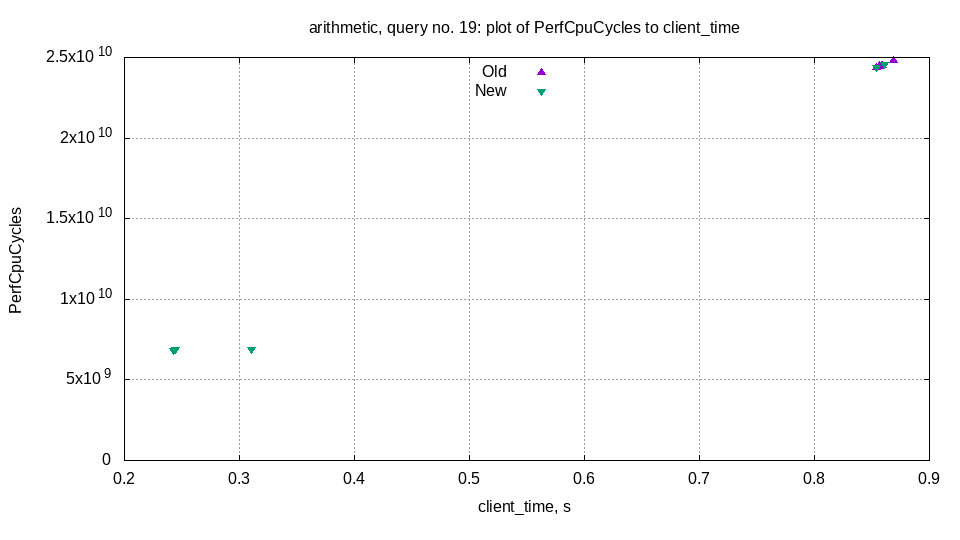## Performance CPU cache misses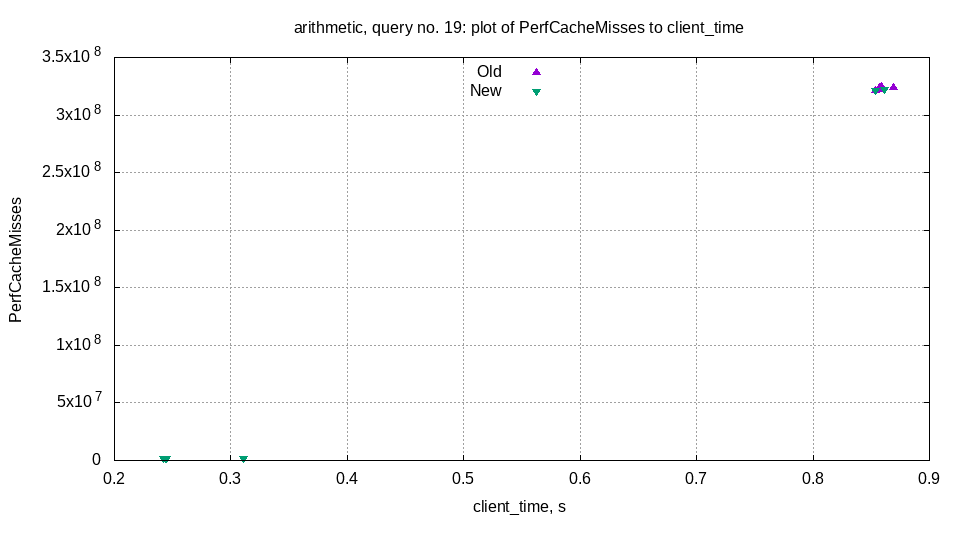## Performance CPU branch misses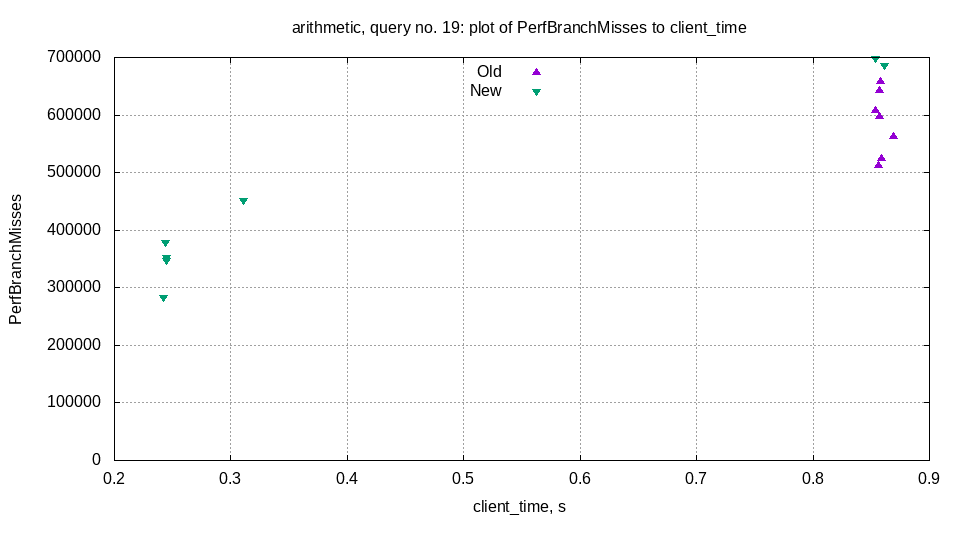## Aggregation in ClickHouse

```EXPLAIN SELECT sum(UserID) FROM default.hits_100m_obfuscated GROUP BY WatchID ┌─explain──────────────────────────────────────┐ │ Expression ((Projection + Before ORDER BY)) │ │ Aggregating │ │ Expression (Before GROUP BY) │ │ SettingQuotaAndLimits │ │ ReadFromMergeTree │ └──────────────────────────────────────────────┘ ```

## Aggregation in ClickHouse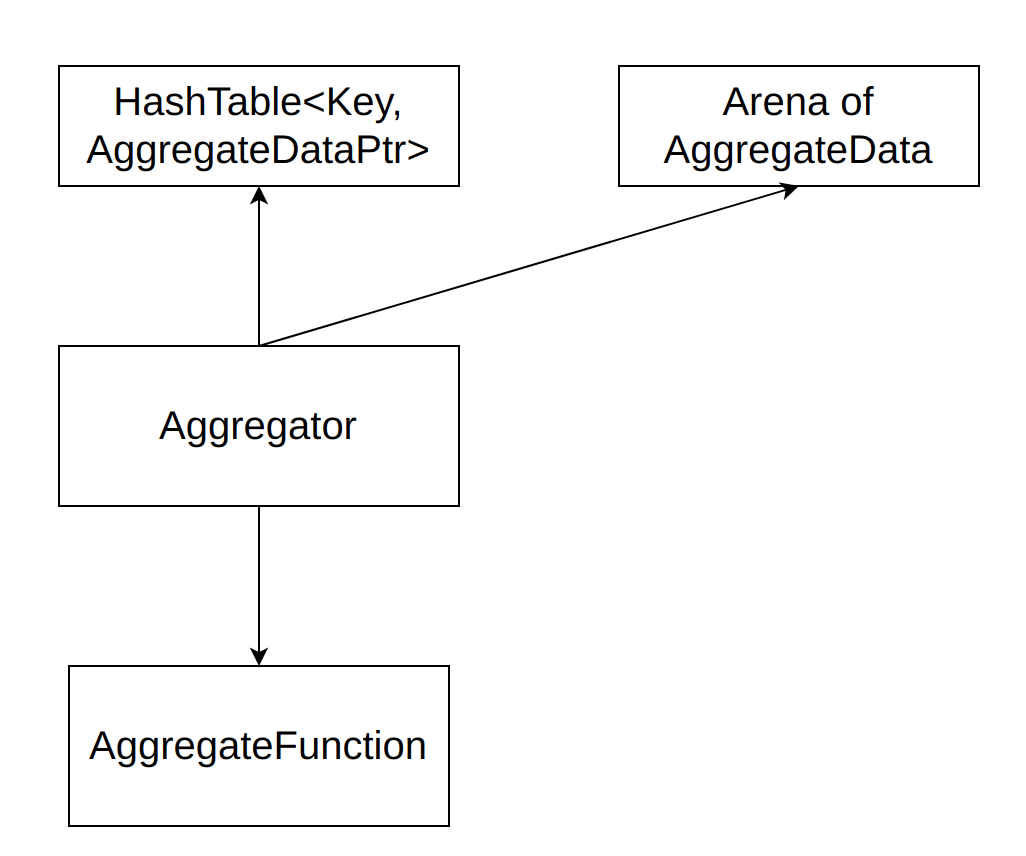## Aggregator interaction with functions

1. Initializaze aggregate data for each function.

2. Fill aggregate data for each function.

3. Merge aggregate data for each function.

4. Insert result into final columns for each function.

1. Nullable wrapper.

2. Aggregation combinators -If, -Array.

Both contain are wrappers around other aggregate function. A lot of indirections.

## JIT compilation examples

```SELECT sum(UserID), sum(ClientIP), sum(CounterClass), sum(CounterID), sum(WatchID) FROM default.hits_100m_obfuscated GROUP BY WatchID ```

— 0 rows in set. Elapsed: 5.504 sec. Processed 100.00 million rows, 2.50 GB (18.17 million rows/s., 454.21 MB/s.)

## JIT compilation examples

```SET compile_aggregate_expression = 1; SELECT sum(UserID), sum(ClientIP), sum(CounterClass), sum(CounterID), sum(WatchID) FROM default.hits_100m_obfuscated GROUP BY WatchID ```

— 0 rows in set. Elapsed: 4.146 sec. Processed 100.00 million rows, 2.50 GB (24.12 million rows/s., 603.06 MB/s.)
+34% performance improvement!

## JIT compilation examples

```SET compile_aggregate_expression = 1; WITH (WatchID % 2 == 0) AS predicate SELECT minIf(UserID, predicate), minIf(ClientIP, predicate), minIf(CounterClass, predicate), minIf(CounterID, predicate), minIf(WatchID, predicate) FROM default.hits_100m_obfuscated GROUP BY WatchID ```

— 0 rows in set. Elapsed: 6.234 sec. Processed 100.00 million rows, 2.50 GB (16.04 million rows/s., 401.05 MB/s.)

## JIT compilation examples

```SET compile_aggregate_expression = 1; WITH (WatchID % 2 == 0) AS predicate SELECT minIf(UserID, predicate), minIf(ClientIP, predicate), minIf(CounterClass, predicate), minIf(CounterID, predicate), minIf(WatchID, predicate) FROM default.hits_100m_obfuscated GROUP BY WatchID ```

— 0 rows in set. Elapsed: 4.146 sec. Processed 100.00 million rows, 2.50 GB (24.12 million rows/s., 603.06 MB/s.)
+71% performance improvement!

## Performance CPU cycles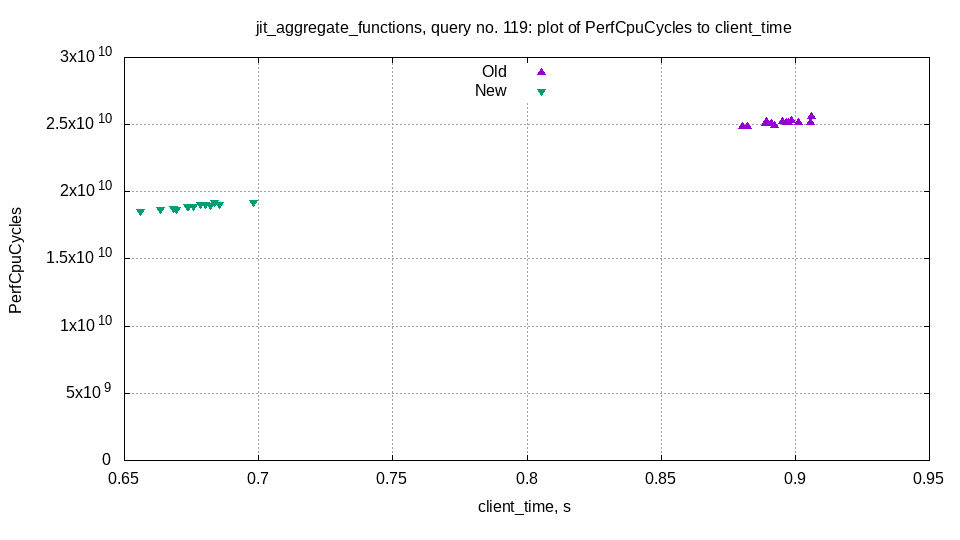## Performance CPU cache misses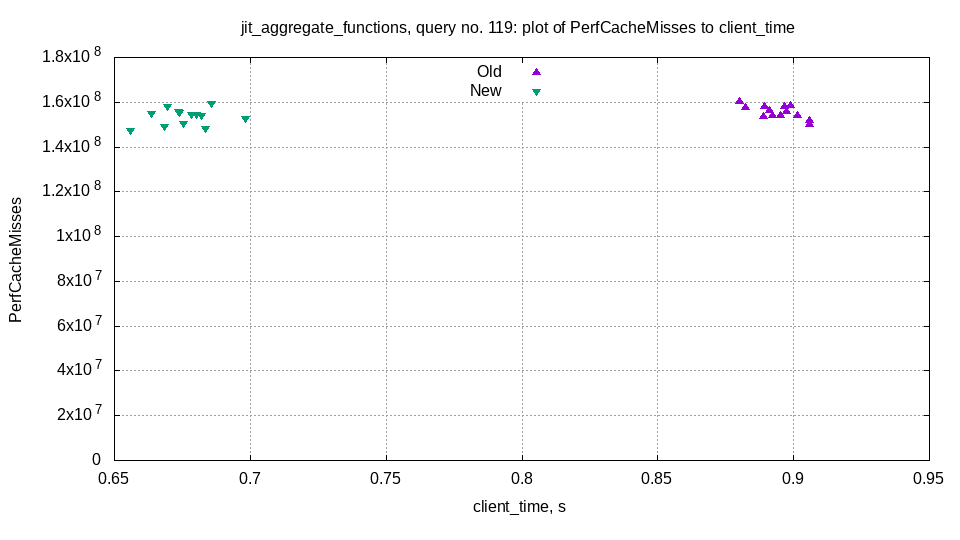## Performance CPU branch misses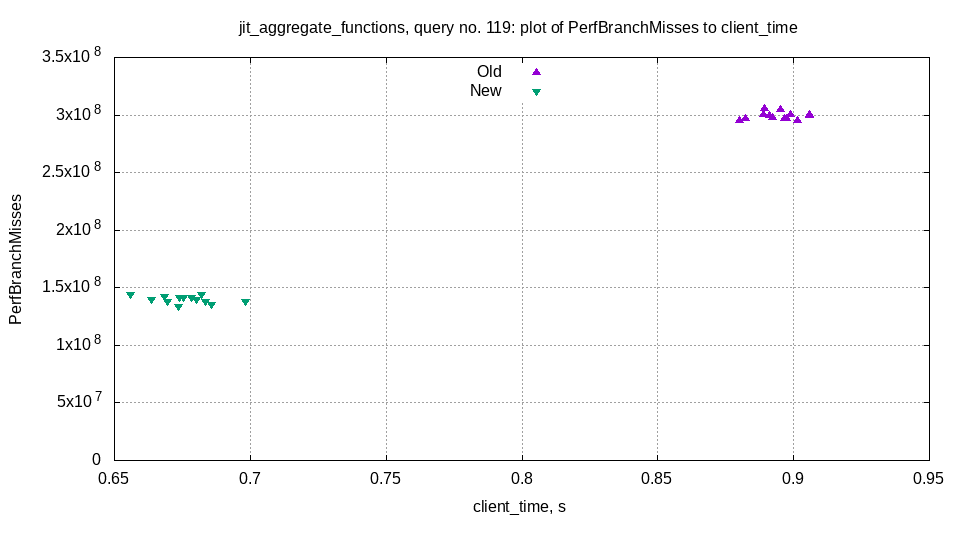## Settings

compile_aggregate_expressions

min_count_to_compile_aggregate_expression by default is 3

compiled_expression_cache_size by default is 1 GB. Same cache with compiled expressions from ExpressionStep.

## Which functions can be compiled

1. Most common aggregate functions sum, count, min, max, avg, avgWeighted

2. Combinators -If